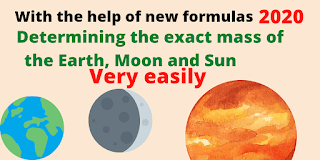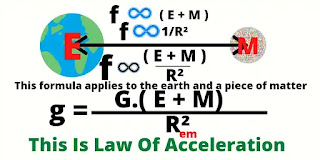# New Law Of Gravitation 2020

Modern law of gravitation 2020 :Modern law of gravitation 2020

Every object in this universe is floating in motion and every two objects attract each other with a force. The central stationary object and orbiting object attract each other with a force which is directly proportional to the product of the of sum of their masses and mass of the orbiting object, and inversely proportional to the square of the radius and it acts along the line joining the masses. This word has to be said differently. The force of attraction between those two objects that is equal to the product of the gravitational acceleration of the stationary object and the mass of the moving object. That's means

### F = m.f = G.(M+m)/R².mModern law of gravitation
This is  a real law of gravitation.

Here, the force of attraction F

Mass of the central stationary object E

Mass of the moving object  M

The radius of the orbit of the orbiting object R

and  Gravitational constants  G

The value of this G is very important for determining the exact mass of the earth.  According to Newton's law, the value of gravitation constant is

Gn = 2л².Lф.r²/T²M

Here, mass of the large ball M = 158kg

Mass of the small ball m = 0.73kg

Length of rod separating small balls L = 1.86m

Separation of large balls L =  1.86m

Distance between centre of the large and small balls r = 0.225m

More recent experiments have used other values

L is the length of the torsion bar ( rod )

r is the equilibrium point distance between M & m

And  T is the oscillation frequency.

The derivative equation for Gn .

Gn = 2л².Lф.r²/T²M = 6.67408÷10⁸ cm³/g.s²

Where, F = G.Mm/R²

According to my formula

F =G.(M+m).m/r²   --------- (1)

So according to my formula ( 1 ) the value of

Gi = 2л².Lф.r²/T²( M+m )

= 6.67408÷10⁸ × 158/(158+0.73) cm³/g.s²

= 6.643385875/10⁸ cm³/gs²

Now we know the value of G.

Here, Gn means Newtonian Gravitational Constant

And Gi is a Ismailian Gravitational Constant,

According to the numberLAW OF ACCELERATION
( 1 ) equation.

Now let's look at the law of gravitational acceleration

## Law Of  Acceleration  :

" Any two particles in this universe attract each other with a force, As a result the moving object moves towards the stationary object with a balanced acceleration. This acceleration is directly proportional the sum of their masses and inversely proportional to the square of the distance between them. In the free state, that acceleration is proportional to their masses."
Now we get the equation of gravitational acceleration. Here, The distance between the Earth and the object R
This Constant G is known as the " Gravitational Constant. "
Gets from this equation
E + M = gR²/G  ------  ( 2 )
We learned the value of G through laboratory tests from the equation no.(1),before. We know the value of this g very well, which is very easy to know with a little experimentation. The avarage value of
g = 981cm/s². Here, weight of M is so small compared to weight of  E that it is ignored. So we get from no. ( 2 ) equation
E = 5.9937 × 10²⁷ grams.
Here,  g = 981 cm/s²
R = ( 6.371 × 10⁸ ) cm
G = ( 6.643385875 ÷ 10⁸) cm³/gs²
So far, we have known the gravitational constant, the gravitational acceleration and the mass of the earth.
Now, let's take a look at some equations at a glance.
1.   F = m.f. here f acceleration.
2.   F = G.(M+m)m/R²
3.  f = w².r = v²/r  here v for velocity
4.  v = 2лr/T  T for  time period
We get from (1) and (2)
G.(M+m)/r² = f    ------  ( 5 )
Get from 5&3  》 G.(M+m)/r² = v²/r.        Or
v²  = G.(M+m)/r --------    (6)
Again,  we get from equation no 4. v² = 4л²r²/T²  --- ( 7)
Now, we get from 6&7
Equation
G.(M+m)/r = 4л². r³/T²
M + m = C. r³/T² --- (10)
Here, C is universal combined Constant.
C=4л²/Gi=5.942514607×10⁸gs²/cm³.
All the equations I have used here are 100% mathematical.
Mass of the moon is 6.3599 × 10²⁵ grams
With this equation, the mass of the sun can be determined very easily and accurately in the same way.
The Sun, like the Moon, moves round the earth in 365.2564 days or 31558149.5 seconds. Here, there is no factor Earth moves round the sun or Sun moves round the Earth. The distance of the sun from the earth is about 1.4959787 × 10¹³ cm. The mass of the sun will be about as much as this 1.9977 × 10³³ grams.
I don't think these mass values ​​are perfect because I think the value of the Earth's gravitational acceleration is higher so I'll discuss them in detail in the next blog post. I hope that my message of truth will reach all over the world in a very short time through this blogger website.